## 梯度下降算法

Posted on Jun 14, 2019

# 模型与假设函数

• m，描述训练样本的数量
• x，描述输入变量或特征
• y，描述输出变量或者叫目标值

x是数据样本的特征，y是其目标值。例如，在预测房价的模型中，x是房子的各种信息，例如：面积，楼层，位置等等，y是房子的价格。在图像识别的任务中，x是图形的所有像素点数据，y是图像中包含的目标对象。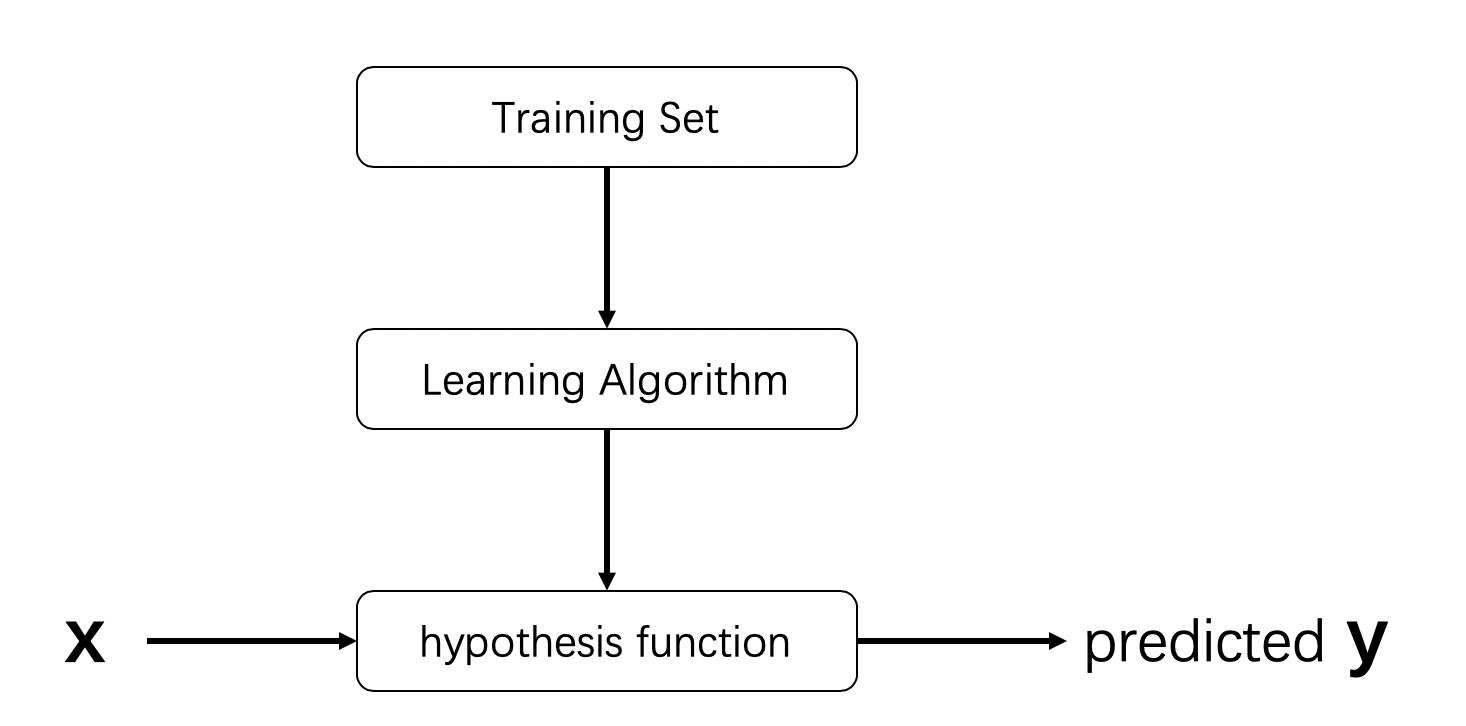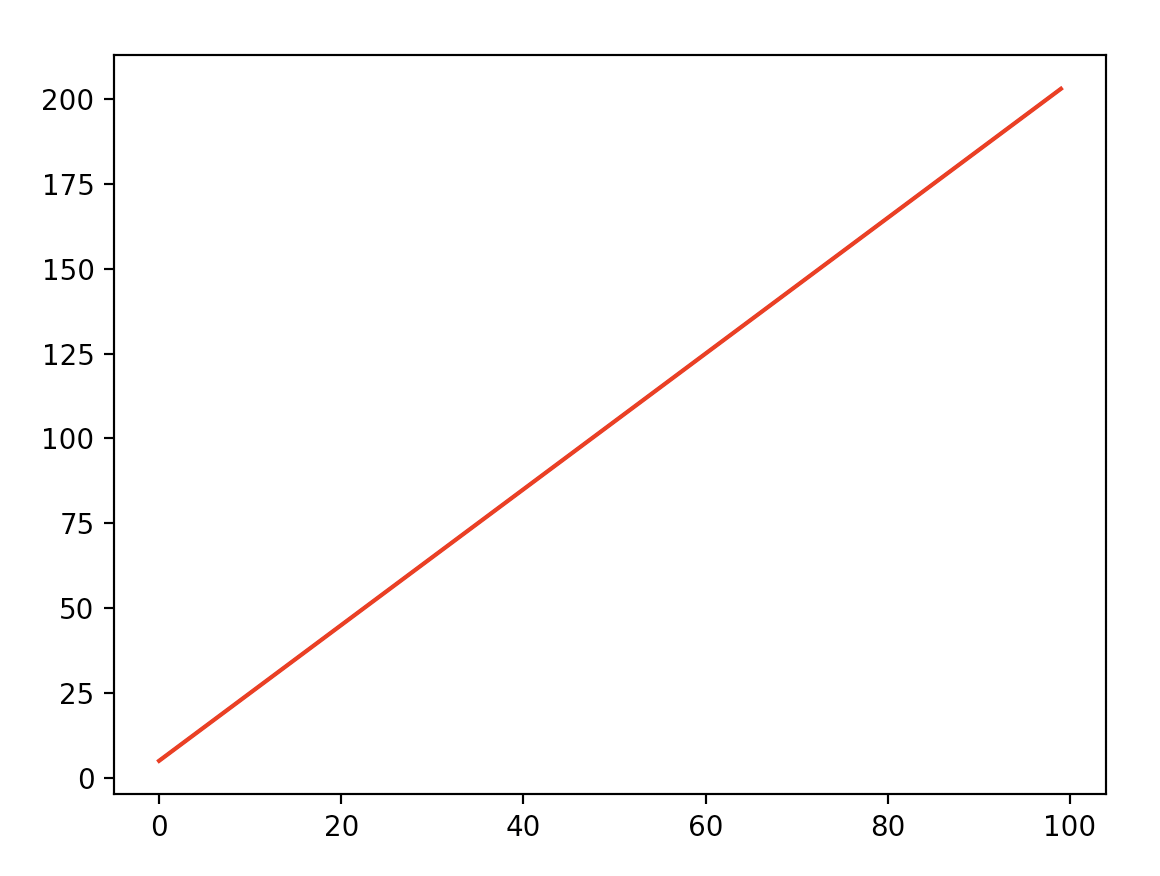# 线性模型与直觉

import numpy as np

max_x = 10
data_size = 10
theta_0 = 5
theta_1 = 2

def get_data():
x = np.linspace(1, max_x, data_size)
noise = np.random.normal(0, 0.2, len(x))
y = theta_0 + theta_1 * x + noise
return x, y


x = [1, 2, 3, 4, 5, 6, 7, 8, 9, 10]
y = [6.66, 9.11, 11.08, 12.67, 15.12, 16.76, 18.75, 21.35, 22.77, 24.56]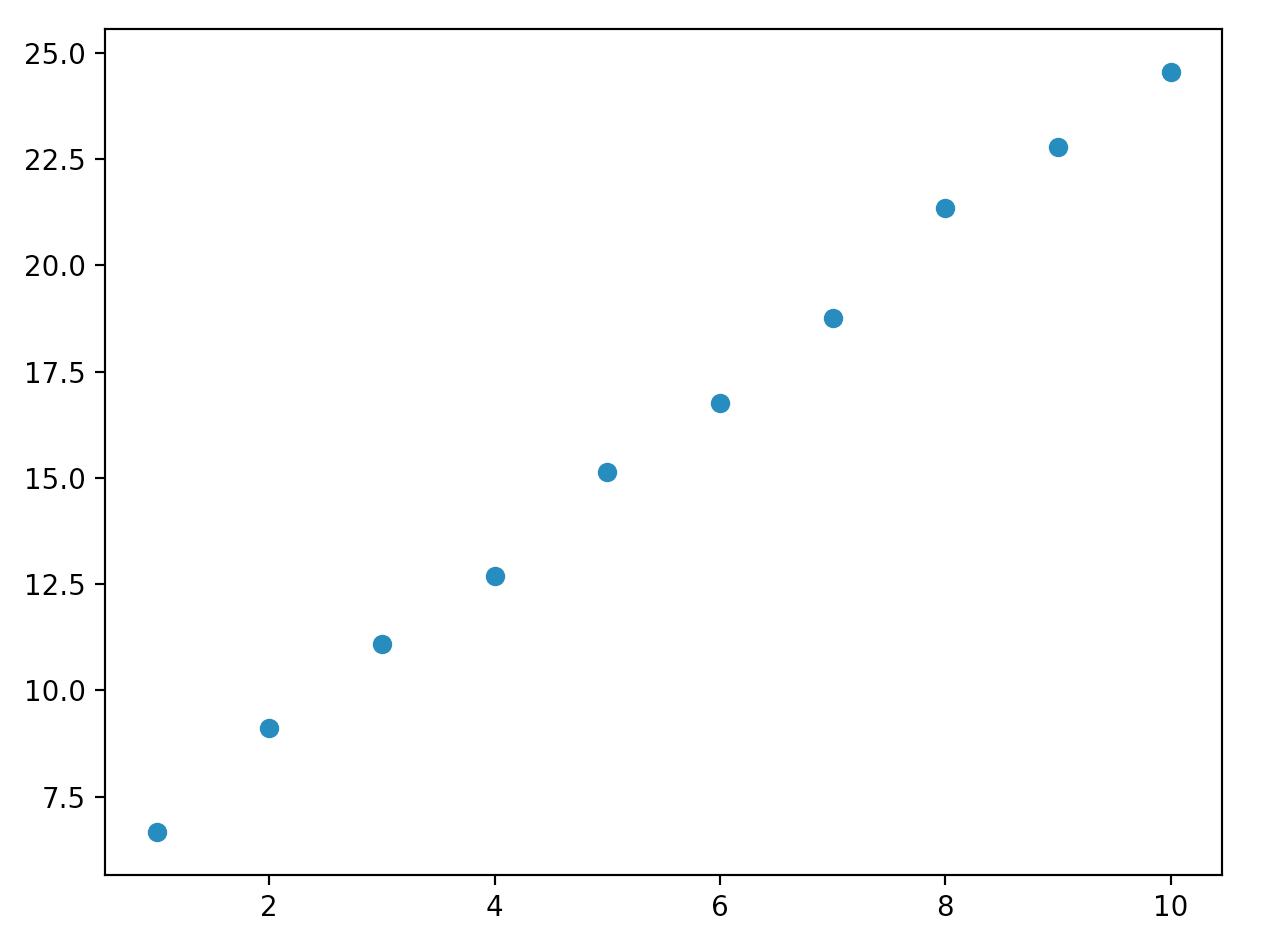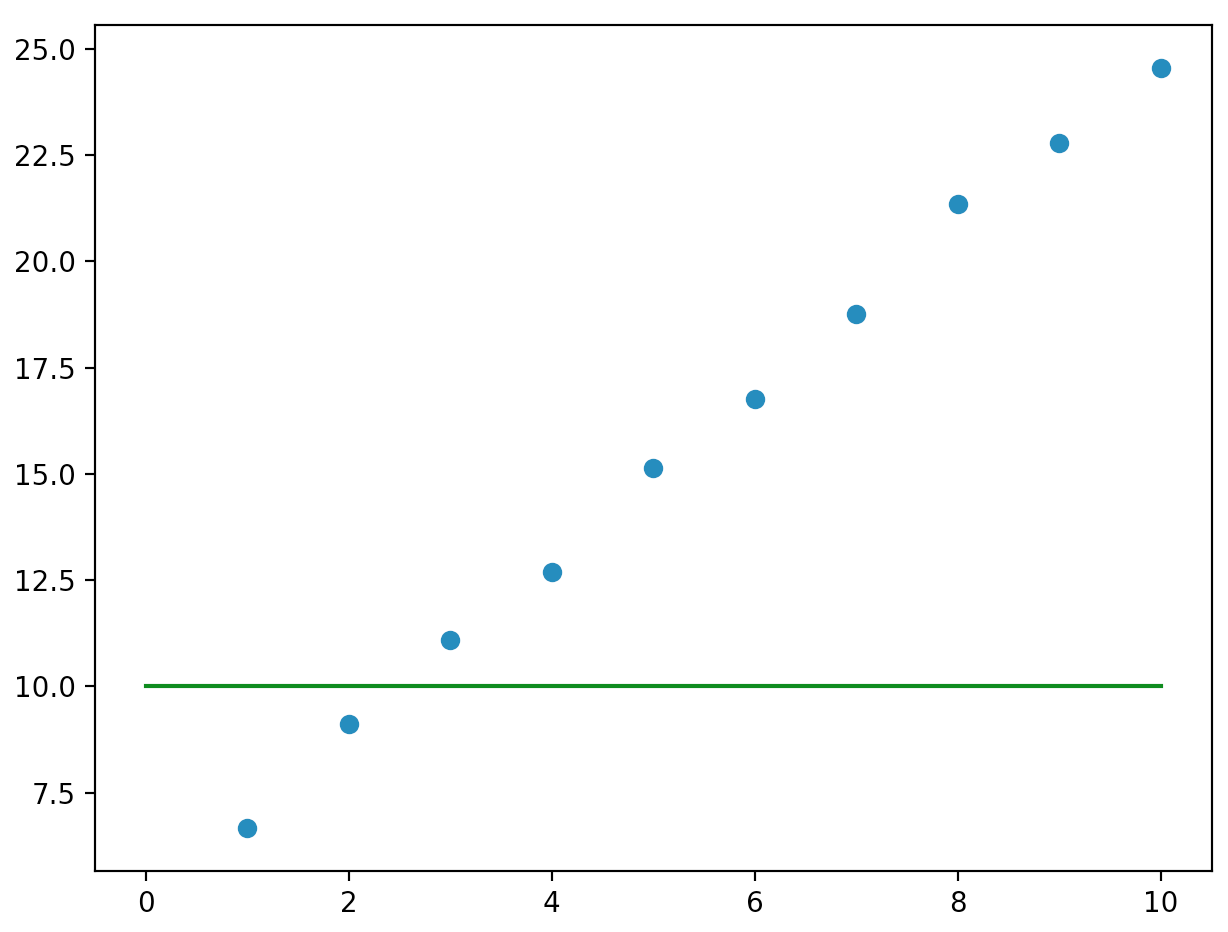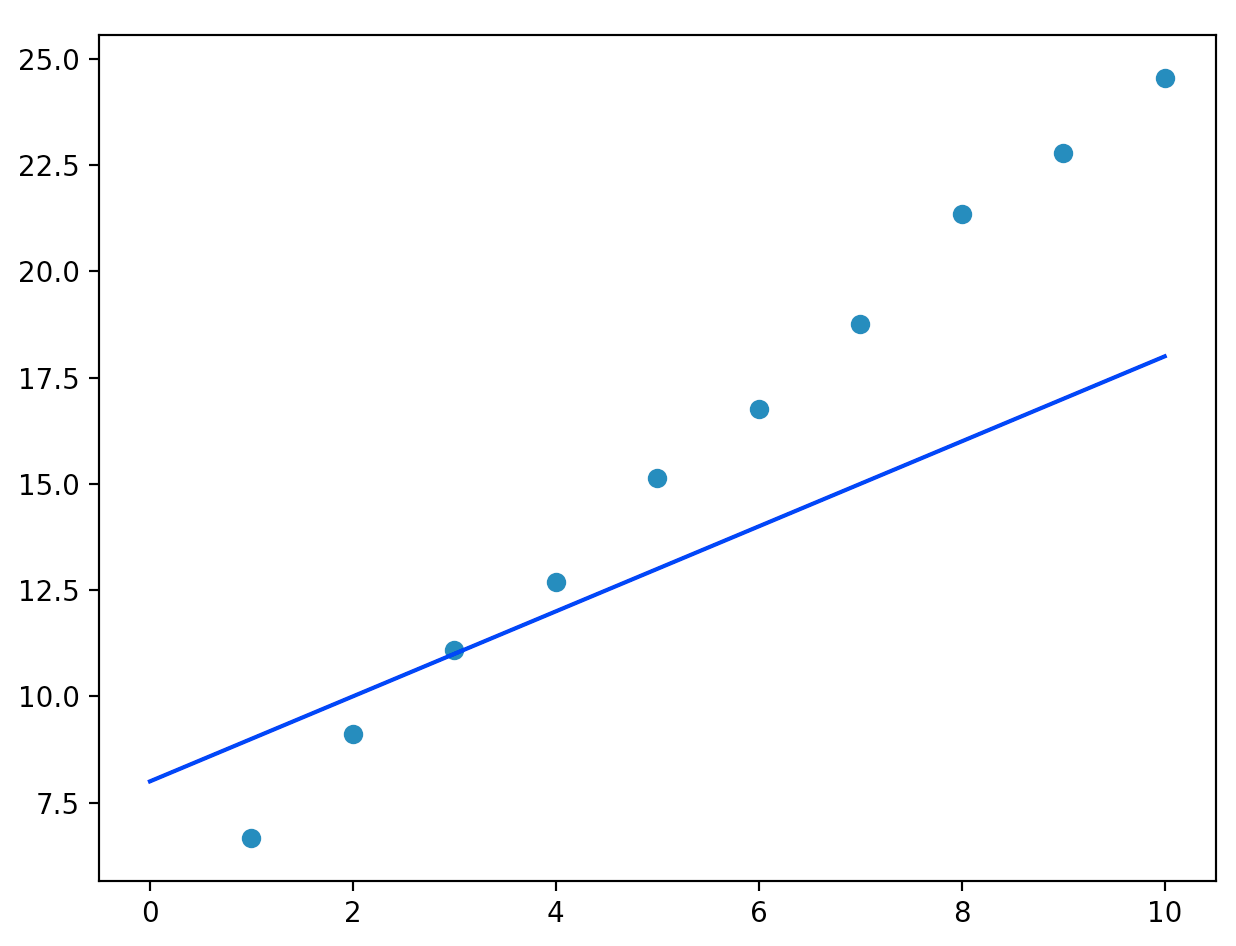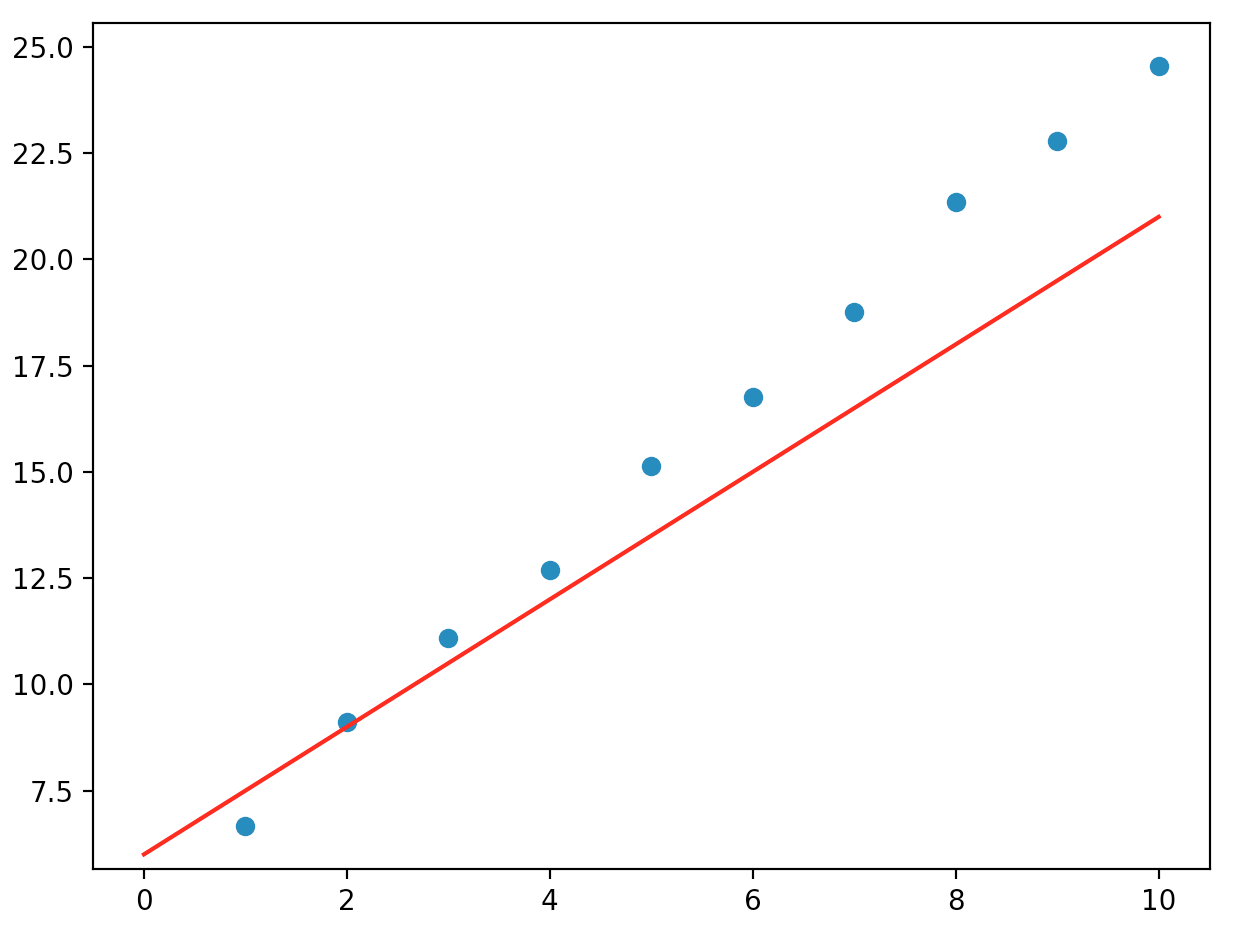# 代价函数

def cost_function(x, y, t0, t1):
cost_sum = 0
for i in range(len(x)):
cost_item = np.power(t0 + t1 * x[i] - y[i], 2)
cost_sum += cost_item
return cost_sum / len(x)


import numpy as np
import matplotlib.pyplot as plt

from matplotlib import cm
from mpl_toolkits.mplot3d import Axes3D

theta_0 = 5
theta_1 = 2

def draw_cost(x, y):
fig = plt.figure(figsize=(10, 8))
ax = fig.gca(projection='3d')
scatter_count = 100
cost = np.zeros((len(t0_range), len(t1_range)))
for a in range(len(t0_range)):
for b in range(len(t1_range)):
cost[a][b] = cost_function(x, y, t0_range[a], t1_range[b])
t0, t1 = np.meshgrid(t0_range, t1_range)

ax.set_xlabel('theta_0')
ax.set_ylabel('theta_1')
ax.plot_surface(t0, t1, cost, cmap=cm.hsv)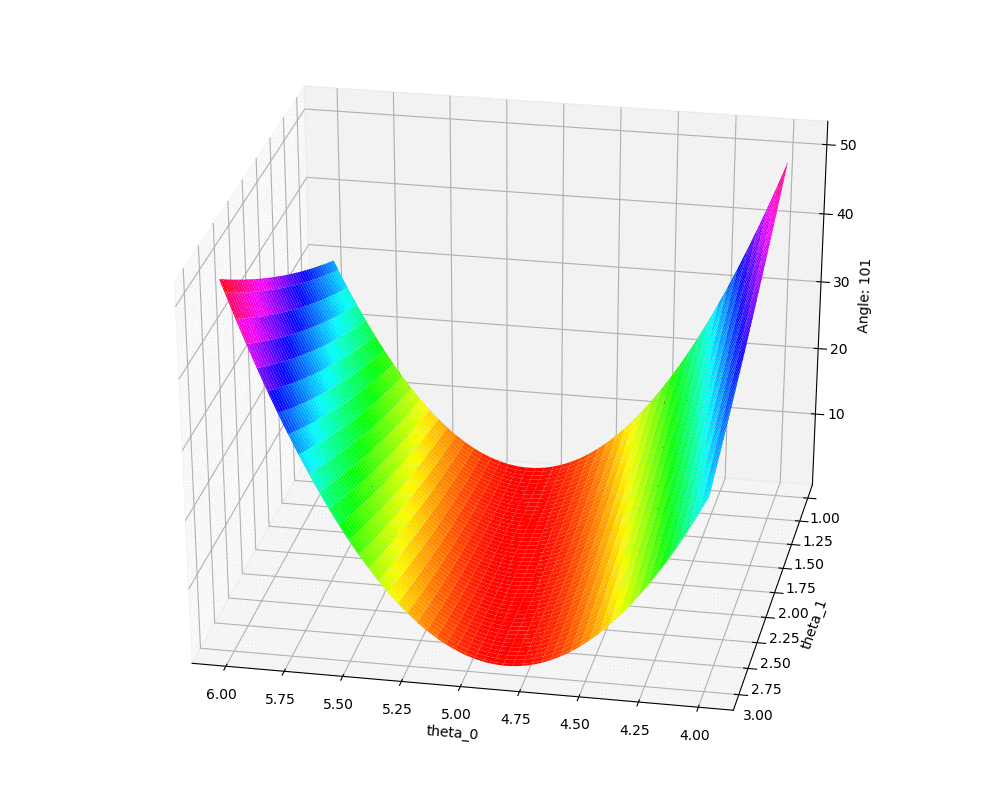# 直观解释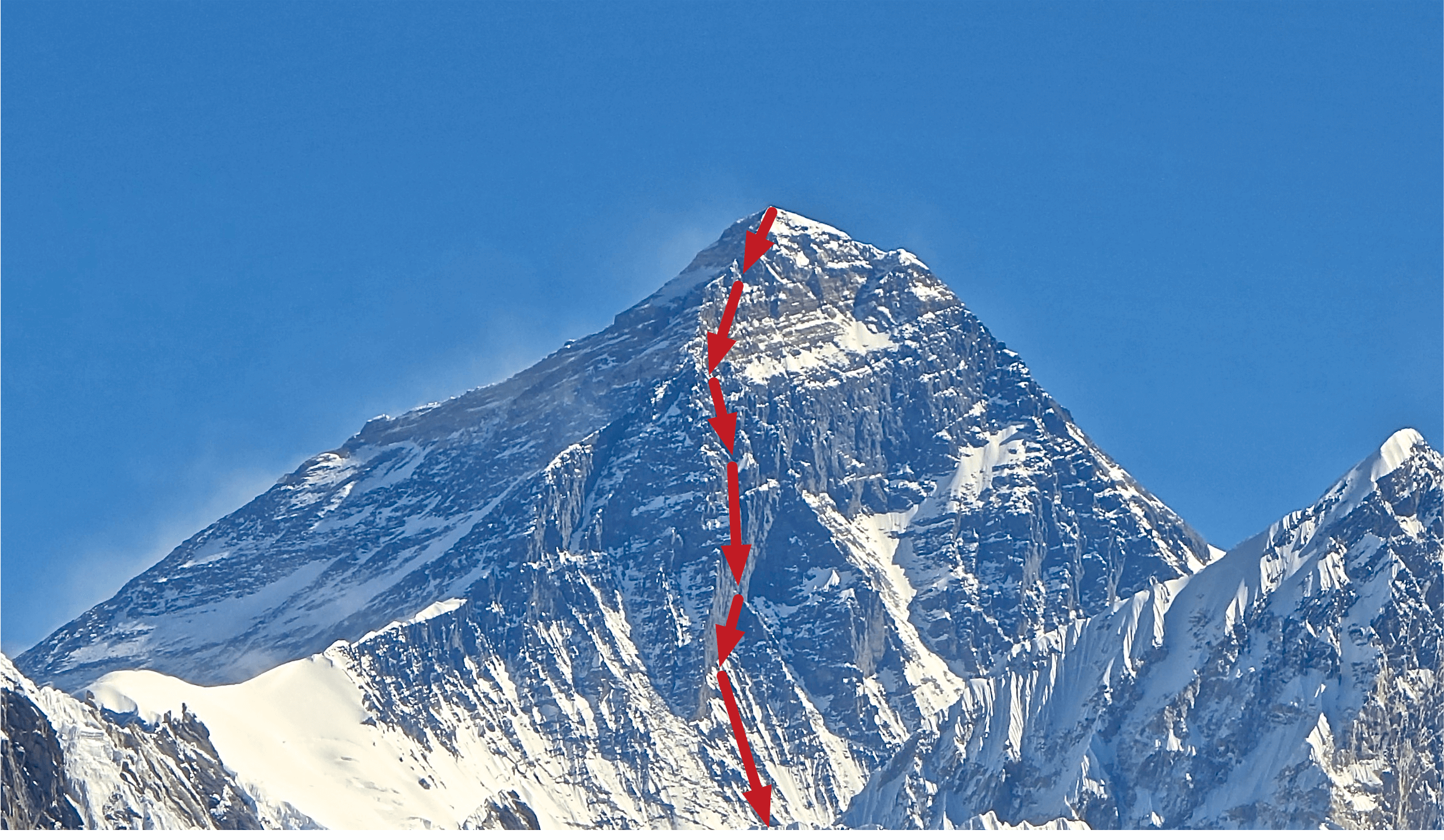• 对于一个函数，怎么确定下行的方向？
• 每一步该往前走多远？
• 有没有可能停留在半山腰的平台上？

# 算法描述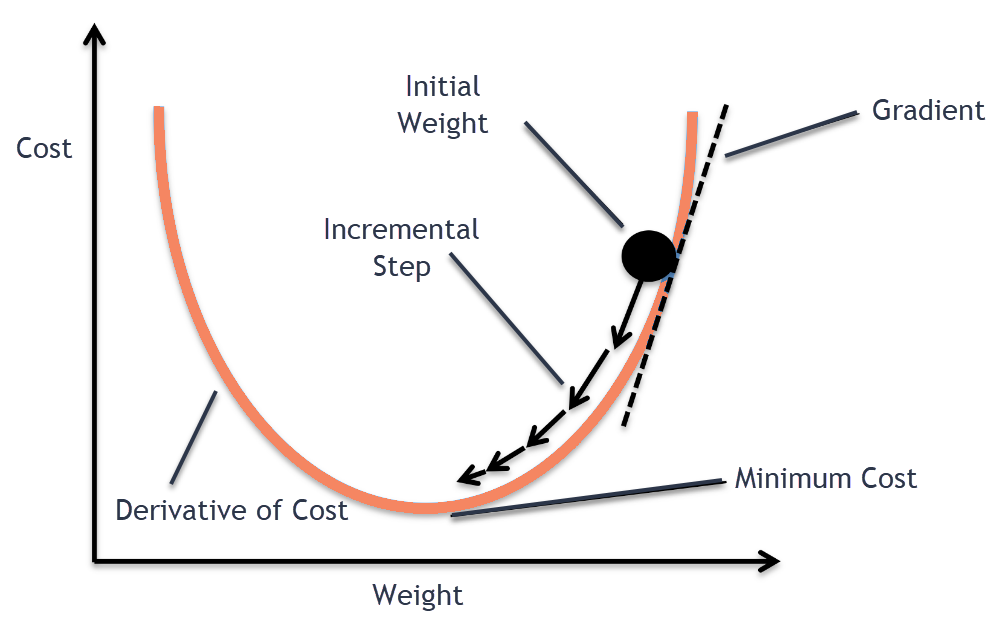1. 收敛是指函数的变化率很小。具体选择多少合适需要根据具体的项目来确定。在演示项目中我们可以选择0.01或者0.001这样的值。不同的值将影响算法的迭代次数，因为在梯度下降的最后，我们会越来越接近平坦的地方，这个时候函数的变化率也越来越小。如果选择一个很小的值，将可能导致算法迭代次数暴增。
2. 公式中的 $\lambda$ 称作步长，也称作学习率（learning rate）。它决定了每一步往前走多远，关于这个值我们会在下文中详细讲解。你可以暂时人为它是一个类似0.01或0.001的固定值。
3. 在具体的项目，我们不会让算法无休止的运行下去，所以通常会设置一个迭代次数的最大上限。

# 线性回归的梯度下降

learning_rate = 0.01

t0 = 10
t1 = 10
delta = 0.001
for times in range(1000):
sum1 = 0
sum2 = 0
for i in range(len(x)):
sum1 += (t0 + t1 * x[i] - y[i])
sum2 += (t0 + t1 * x[i] - y[i]) * x[i]
t0_ = t0 - 2 * learning_rate * sum1 / len(x)
t1_ = t1 - 2 * learning_rate * sum2 / len(x)
print('Times: {}, gradient: [{}, {}]'.format(times, t0_, t1_))
if (abs(t0 - t0_) < delta and abs(t1 - t1_) < delta):
return t0_, t1_
t0 = t0_
t1 = t1_
return t0, t1


• 我们随机选择了 $\theta_{0}, \theta_{1}$ 都为10作为起点
• 设置最多迭代1000次
• 收敛的范围设为0.001
• 学习步长设为0.01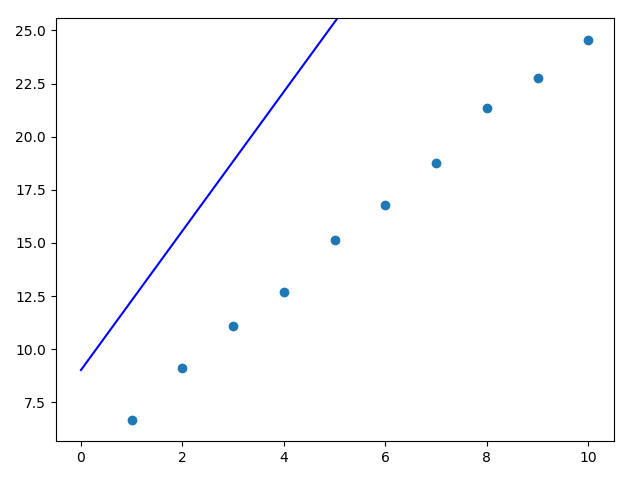...


# 梯度下降家族

## MBGD

• 如果样本数量较小（例如小于等于2000），选择BGD即可。
• 如果样本数量很大，选择$2^n$ 来进行MBGD，例如：64，128，256，512。

BGD
SGD 好（with annealing）
MBGD 中等 中等

# 算法优化

## Momentum

Momentum是动量的意思。这个算法的思想就是借助了动力学的模型：每次算法的迭代会使用到上一次的速度作为依据。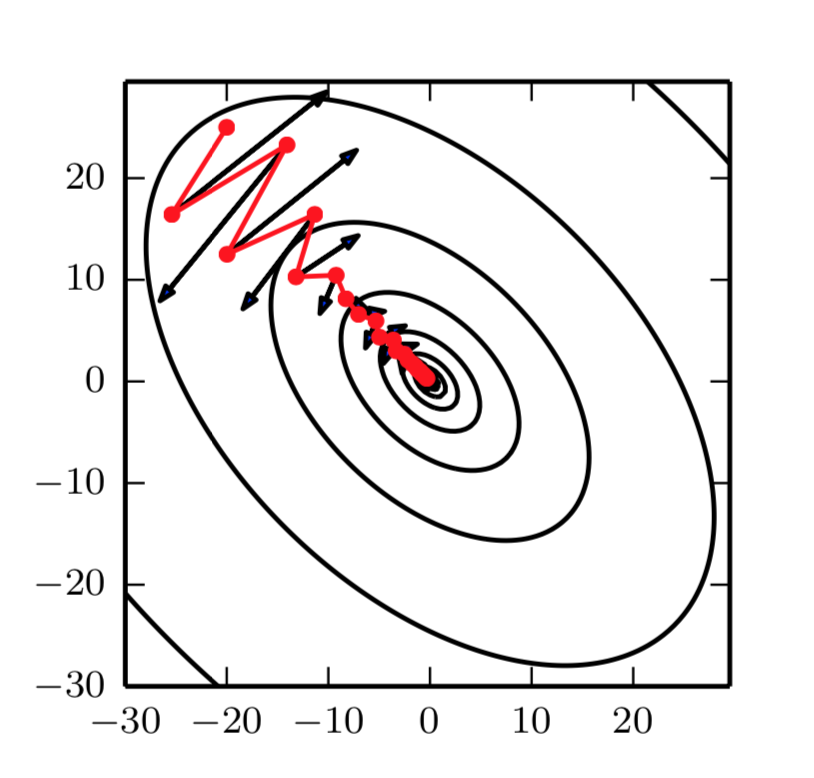def gradient_descent_with_momentum(x, y):
t0 = 10
t1 = 10
delta = 0.001
v0 = 0
v1 = 0
gamma = 0.9
for times in range(1000):
sum1 = 0
sum2 = 0
for i in range(len(x)):
sum1 += (t0 + t1 * x[i] - y[i])
sum2 += (t0 + t1 * x[i] - y[i]) * x[i]
v0 = gamma * v0 + 2 * learning_rate * sum1 / len(x)
v1 = gamma * v1 + 2 * learning_rate * sum2 / len(x)
t0_ = t0 - v0
t1_ = t1 - v1
print('Times: {}, gradient: [{}, {}]'.format(times, t0_, t1_))
if (abs(t0 - t0_) < delta and abs(t1 - t1_) < delta):
return t0_, t1_
t0 = t0_
t1 = t1_
return t0, t1


...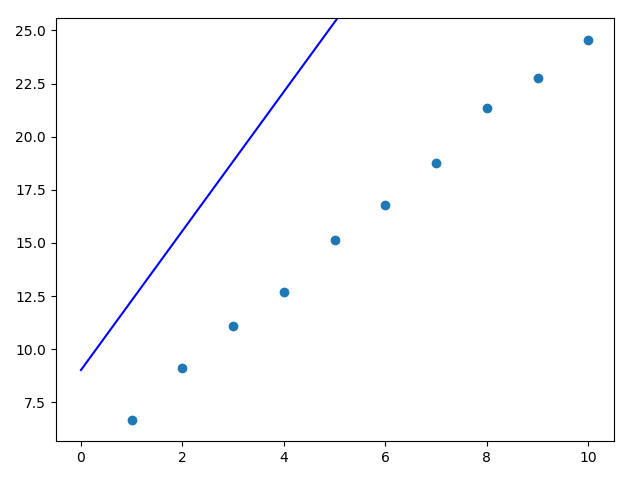## Learning Rate 优化

《深度学习》一书中是这样描述的：“与其说是科学，这更像是一门艺术，我们应该谨慎地参考关于这个问题的大部分指导。”。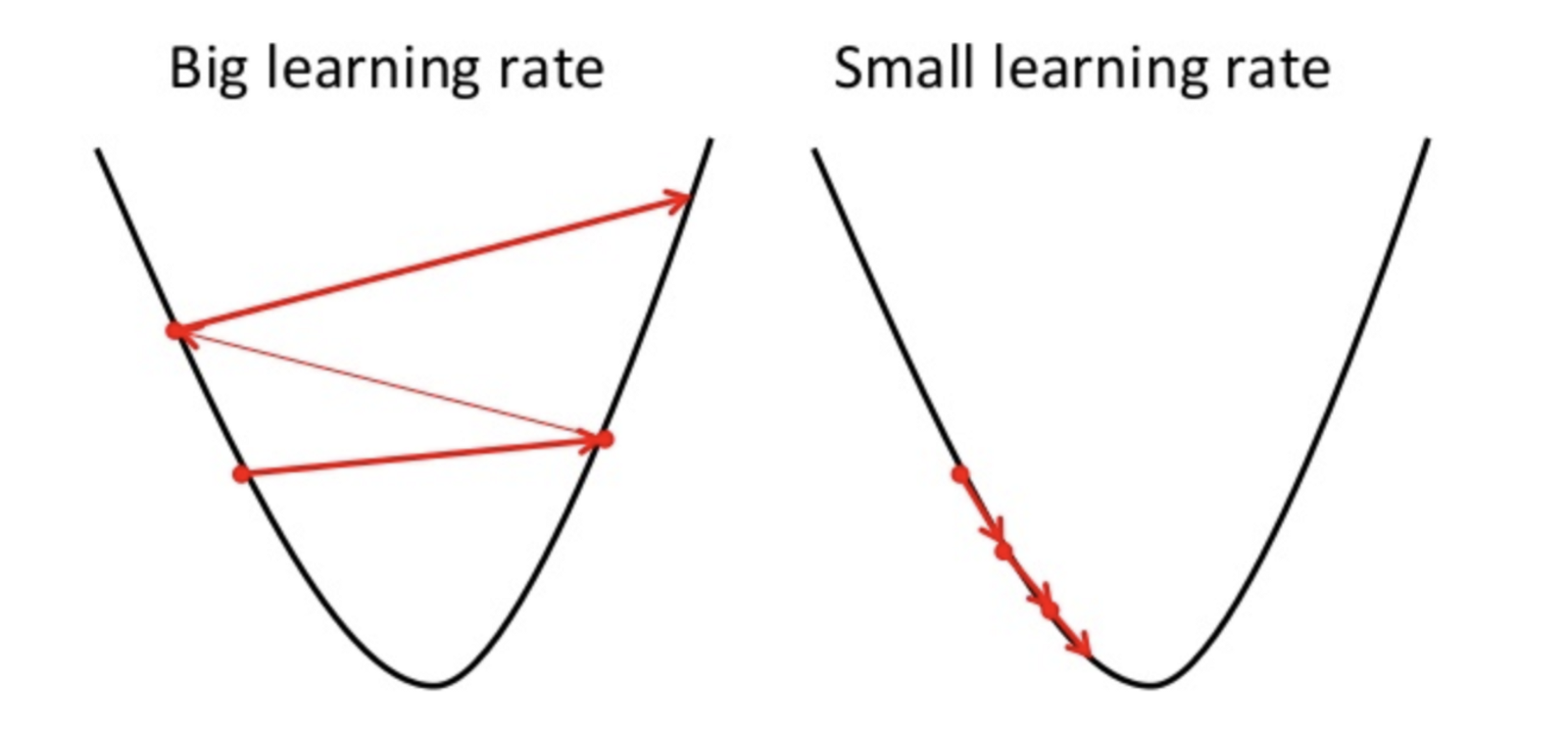AdaGrad是Adaptive Gradient的简写，该算法会为每个参数$\theta_{i}$设定不同的学习率。它使用历史梯度的平方和作为基础来进行计算。

### RMSProp

$m^{t}$，$m^{t}$分别是对梯度的一阶矩估计和二阶矩估计。$\widehat{m}^{t}$，$\widehat{v}^{t}$ 是对$m^{t}$，$m^{t}$的校正，这样可以近似为对期望的无偏估计。

Adam算法的提出者建议$\beta_{1}$ 默认值为0.9，$\beta_{2}$默认值为0.999，$\epsilon$默认值为 $10^{-8}$。

# 算法限制

## 局部最小值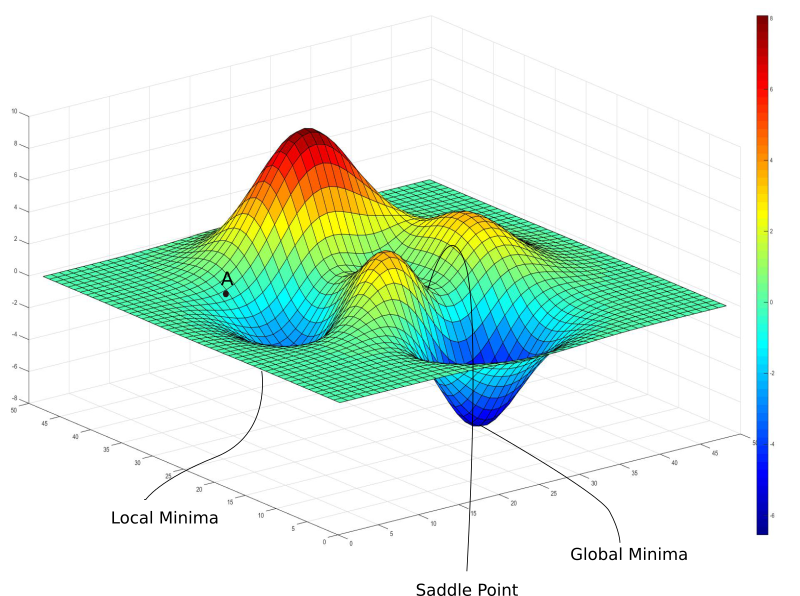1. 对于凸函数或者凹函数来说，不存在局部极值的问题。其局部极值一定是全局极值。
2. 最近的一些研究表明，某些局部极值并没有想象中的那么糟糕，它们已经非常的接近全局极值所带来的结果了。

## 鞍点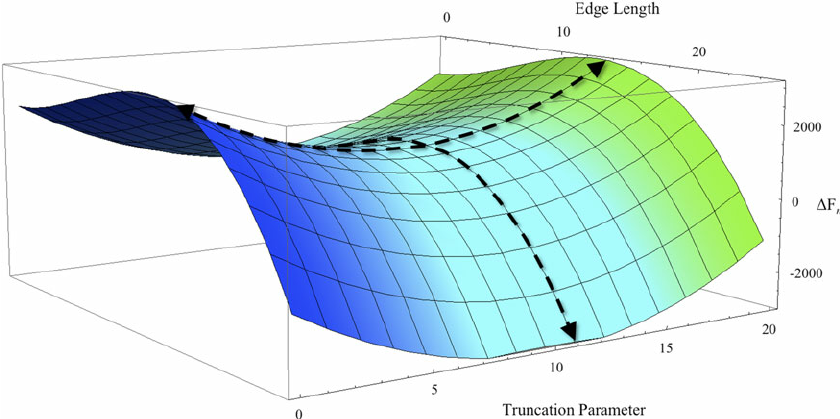Contents